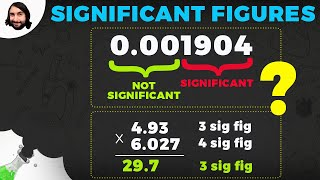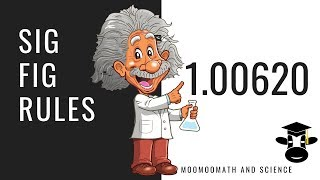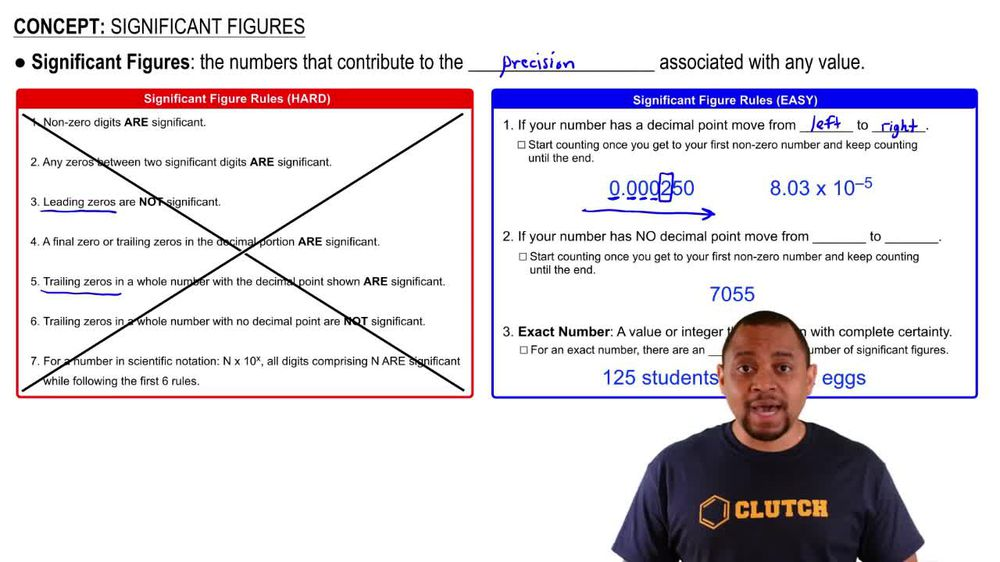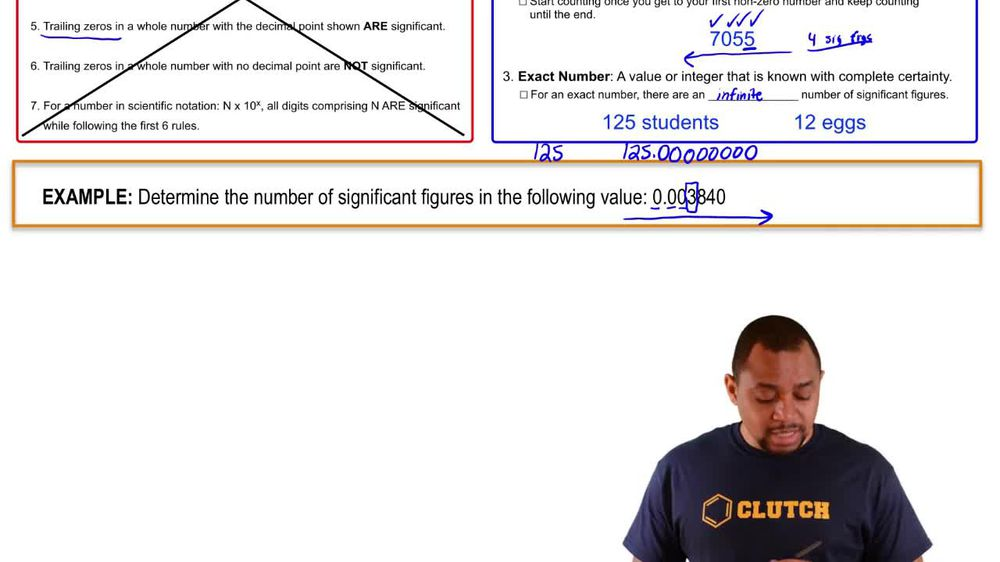Start typing, then use the up and down arrows to select an option from the list.
1. 1. Intro to General Chemistry2. Significant Figures
Problem

# Indicate which of the following are exact numbers: (a) the mass of a 3- by 5-in. index card, (b) the number of ounces in a pound, (c) the volume of a cup of Seattle’s Best coffee, (d) the number of inches in a mile, (e) the number of microseconds in a week, (f) the number of pages in this book.

Relevant Solution2m
Play a video:
Hey there, welcome back. Alright. So here we're going to be selecting the exact numbers and the following. So an exact number has basically we know those numbers with complete certainty. Right? So there is complete certainty with those numbers um as long as you know, as long as their exact numbers and these are numbers that are not measured. If anything that's measured length, volume mass um anything that's measured, obviously it will be an an exact number because it will have some sort of uncertainty to it. Alright, so massive, a black black ball pen. So its mass. You have to measure this. So it's never going to be an exact number, the average height of buildings around the park. So first of all, height of the building has to be measured. So it's not going to be exact and because it's an average average will never give you an exact number. So no, the number of leaders in the cubic feet of water. So let's talk about this. So um leaders equals two however many cubic feet of water. That's a conversion factor is the same thing as saying, you know, like let's say one millimeter Right, which is length equals to one cm cubed. Right? So here you're converting length into volume. So this is a conversion factor, the same as a number of leaders in the cubic feet. You're not actually measuring this conversion factor, you're using that to convert a given number to a different number or in different units. Right, So this one is actually an exact number, it will never change. It's always going to be the same number of millimeters and one centimeter cubed or the same number of leaders in a certain number of cubic feet of water. That will never change. So it's always going to be exact the number of employees in an office. I think this one is pretty obvious. This is an exact number, right? Because you can just count the number of employees, you know exactly how many there are. You can't have like, you know, 10.5 or 0.1 people. It's always going to be an exact number. As long as, you know, as long as it's not an average, it's an average of people. Obviously that's not an exact, but this is just a number and then the mass again, we're talking about mass here. So no, you have to weigh, you have to measure that. That will never be exact. There's always going to be some level of uncertainty. So cnd here are the answers. Thank you so much for watching